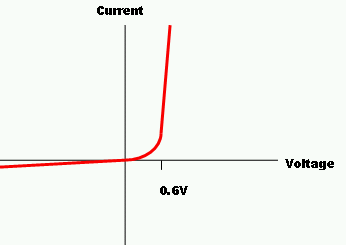# Limitations of Ohm’s Law

## Limitations of Ohm’s Law

Although Ohm’s law is an important principle in the field of electronics, it cannot be considered as an actual physical law. We know that as per ohm’s law voltage / current = a constant and that constant is the resistance. But the resistance does not remain constant all the time. When the current passes through a material, it heats up. When the temperature of the material changes, the resistance changes. So when the resistance changes, and we divide the voltage across the resistor by the current passing through it, we will not get the same numbers always.

Ohm’s law is applicable and valid for many materials. But there are some materials that do not support the ohm’s law. Some materials and devices which are used in electric circuits do not have the proportionality between voltage and current. For Example, consider a diode and plot a graph between voltage and current. When the value of voltage is marked in the reverse direction by keeping the magnitude fixed, it produces the current with different magnitude in the opposite direction. The relationship between the voltage and current is not linear.Diodes

For Example when we p- n junction diode is forward biased, initially the current rises slowly even though the voltage increases and the current rises rapidly. Non – linear elements do not support ohm’s law. Thyristor and electric arc are examples for this. Materials like Ga As produces more than one value of V for the same value of current.Diode do not support Ohm’s law

Consider a water voltameter. Here although the voltage is increased the current increases only after a certain value of voltage. Ohm’s law is not applicable for unilateral networks. The conductors which does not obey ohm’s law is called Non – Ohmic Conductors. Semi – conductors like Germanium and silicon do not obey Ohm’s law. Other examples include transistor, vacuum tubes, diode and triode valve. The circuits which consists of non - ohmic conductors are known as Non – Ohmic Circuits. Ohm’s law is valid only when the temperature and other physical parameters do not affect the resistance of the metals conductors.

Another example is incandescent bulb. The tungsten filament which is present in the bulb is heated up when the voltage increases, the resistance of the wire changes. So the bulb filament does not support ohm’s law. The law is not applied for LED, which is a light emitting diode.

## Summary

• George Simon Ohm stated the Ohm’s law. He stated that the electric current flowing through a wire will be directly proportional to the potential difference across the ends when the temperature remains constant. That constant is the resistance. V = I R

• As per ohm’s law voltage / current = a constant. The resistance of a material changes when the temperature changes. So when the resistance changes, the ratio of the voltage across the resistor and the current passing through it will not be a constant. We will not get the same numbers always.

• Non – linear elements and unilateral networks do not support ohm’s law. Examples are diodes, transistor etc. The conductors which does not obey ohm’s law is called Non – Ohmic Conductors. Incandescent bulb do not support ohm’s law.

Watch this Video for more reference

Limitations of Ohm’s Law### Course Features

• Video Lectures
• Revision Notes
• Previous Year Papers
• Mind Map
• Study Planner
• NCERT Solutions
• Discussion Forum
• Test paper with Video Solution